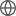Old Web
English
# A Unicorn in Monoceros: the $3M_\odot$ dark companion to the bright, nearby red giant V723 Mon is a non-interacting, mass-gap black hole candidate
We report the discovery of the closest known black hole candidate as a binary companion to V723 Mon. V723 Mon is a nearby ($d\sim460\,\rm pc$), bright ($V\simeq8.3$~mag), evolved ($T_{\rm eff, giant}\simeq4440$ K, $L_{\rm giant}\simeq173~L_\odot$ and $R_{\rm giant}\simeq22 ~R_\odot$) red giant in a high mass function, $f(M)=1.72\pm 0.01~M_\odot$, nearly circular binary ($P=59.9$ d, $e\simeq 0$). V723 Mon is a known variable star, previously classified as an eclipsing binary, but its ASAS, KELT, and TESS light curves are those of a nearly edge-on ellipsoidal variable. Detailed models of the light curves constrained by the period, radial velocities and stellar temperature give an inclination of $i=87.0^\circ \pm 1.0^\circ$, a mass ratio of $q\simeq0.30\pm0.02$, a companion mass of $M_{\rm comp}=2.91\pm0.08~M_\odot$, a stellar radius of $R_{\rm giant}=23.6\pm1.0~R_\odot$, and a giant mass of $M_{\rm giant}=0.87\pm0.08~ M_\odot$. We identify a likely non-stellar, diffuse veiling component with contributions in the $B$ and $V$-band of ${\sim}64\%$ and ${\sim}23\%$, respectively. The SED and the absence of continuum eclipses imply that the companion mass must be dominated by a compact object. We do observe eclipses of the Balmer lines when the dark companion passes behind the giant, but their velocity spreads are low compared to observed accretion disks. The X-ray luminosity of the system is $L_{\rm X}\simeq1.0\times10^{30}~\rm ergs~s^{-1}$, corresponding to $L/L_{\rm edd}{\sim}10^{-9}$.The simplest explanation for the massive companion is a single compact object, most likely a black hole in the "mass gap", although a double neutron star binary is possible.